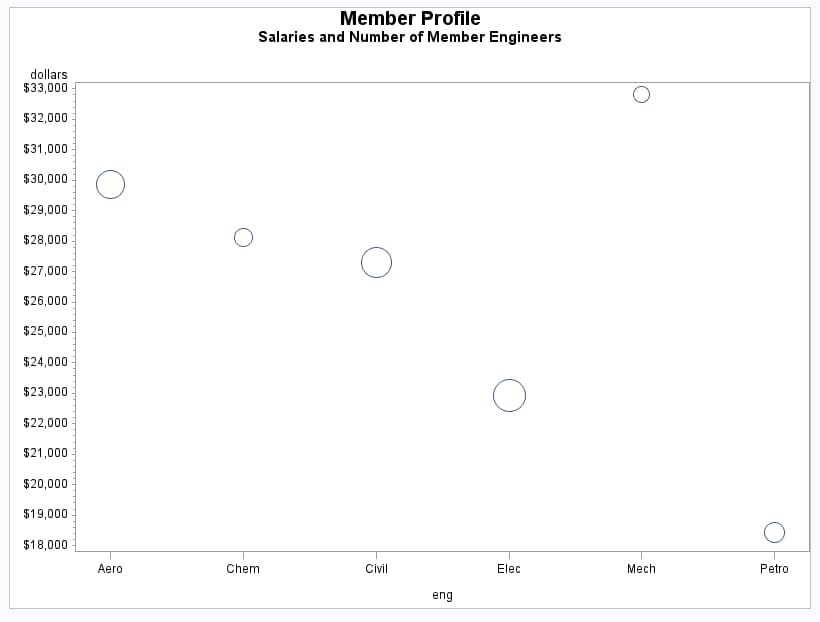# Producing Two-Dimensional Plots

Plots are useful for demonstrating the relationship between two or more variables and frequently compare trends or data values or depict movements of data values over time. SAS/GRAPH uses the GPLOT procedure to produce two-dimensional graphs that plot one or more dependent variables against an independent variable within a set of coordinate axes. The GPLOT procedure can display the data points as individual symbols (as in a scatter plot). It can also use interpolation methods specified by the SYMBOL statement to join the points. You can request spline interpolation or regression analysis, produce various high-low charts, or generate several other types of plots. The GPLOT procedure can also display data as bubble plots in which circles of different sizes represent the values of a third variable. See GPLOT Procedure for more information.

## Scatter Plots

Two-dimensional scatter plots show the relationship of one variable to another, often revealing concentrations or trends in the data. Typically, each variable value on the horizontal axis can have any number of corresponding values on the vertical axis.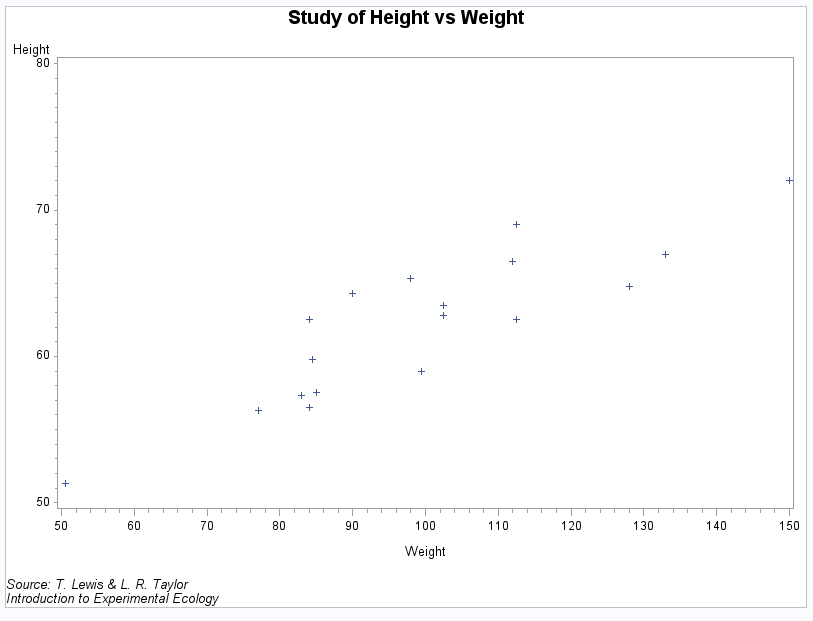## Simple Line Plots

Simple line plots show the relationship of one variable to another, often as movements or trends in the data over a period of time. Typically, each variable value on the horizontal axis has only one corresponding value on the vertical axis. The line connecting data points can be smoothed using a variety of interpolation methods, including the Lagrange and the cubic spline interpolation methods.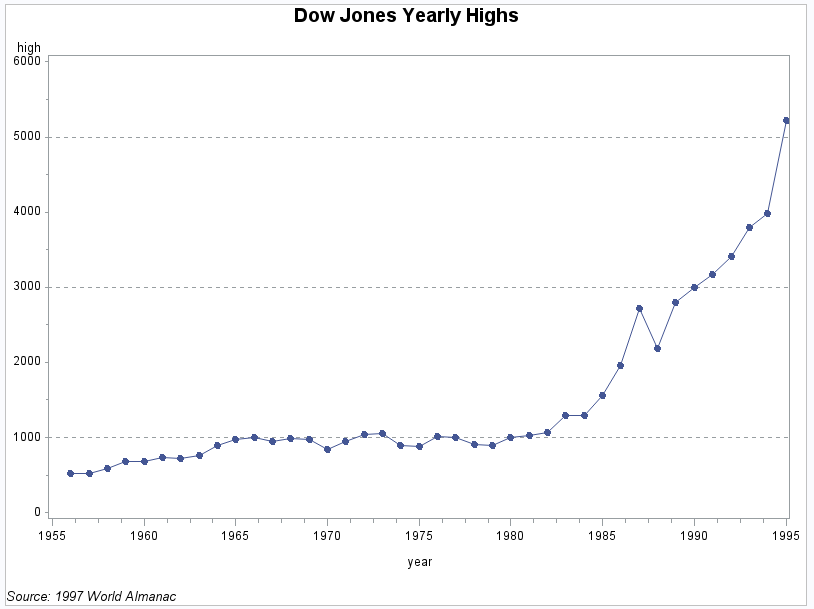## Regression Plots

Regression plots specify that the plot is a regression analysis. You can specify one of three types of regression equation: linear, quadratic, or cubic. You can choose to display confidence limits for mean predicted values or individual predicted values.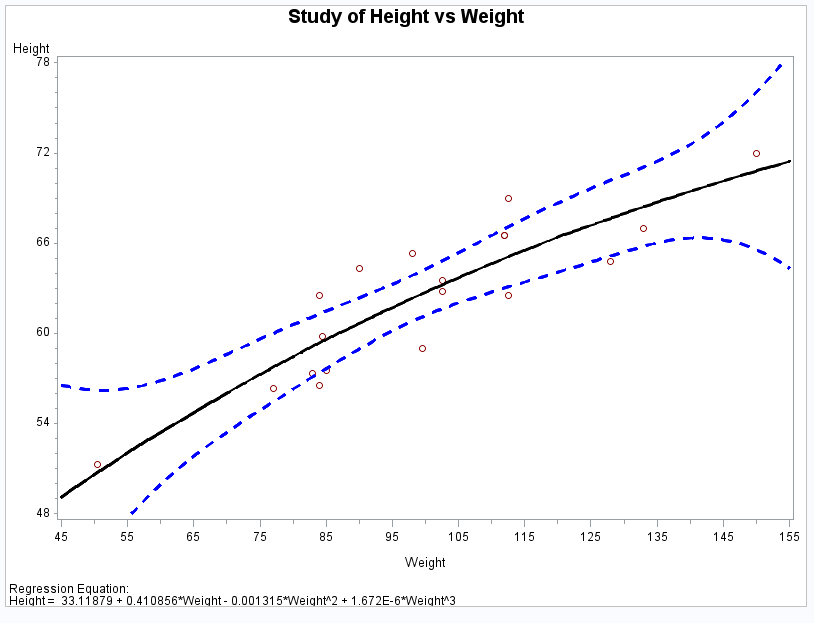## High-Low Plots

High-low plots show how several values of one variable relate to one value of another variable. Typically, each variable value on the horizontal axis has several corresponding values on the vertical axis. High-low plots include box, needle, and stock market plots.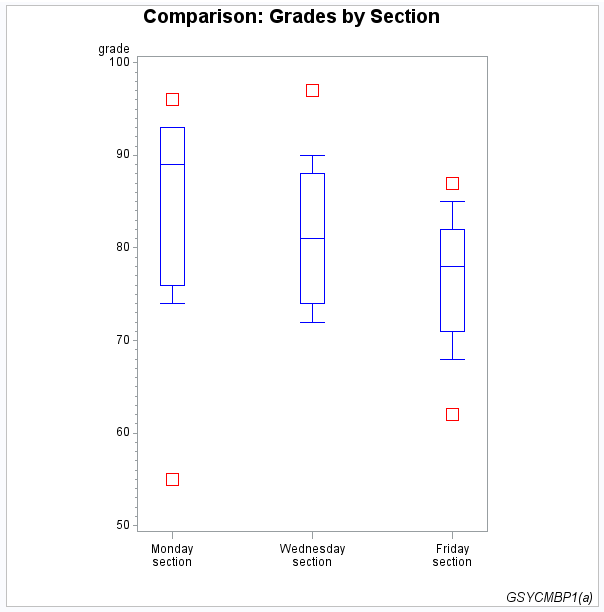## Bubble Plots

Bubble plots show the relative magnitude of one variable in relation to two other variables. The values of two variables determine the position of the bubble on the plot, and the value of a third variable determines the size of the bubble.